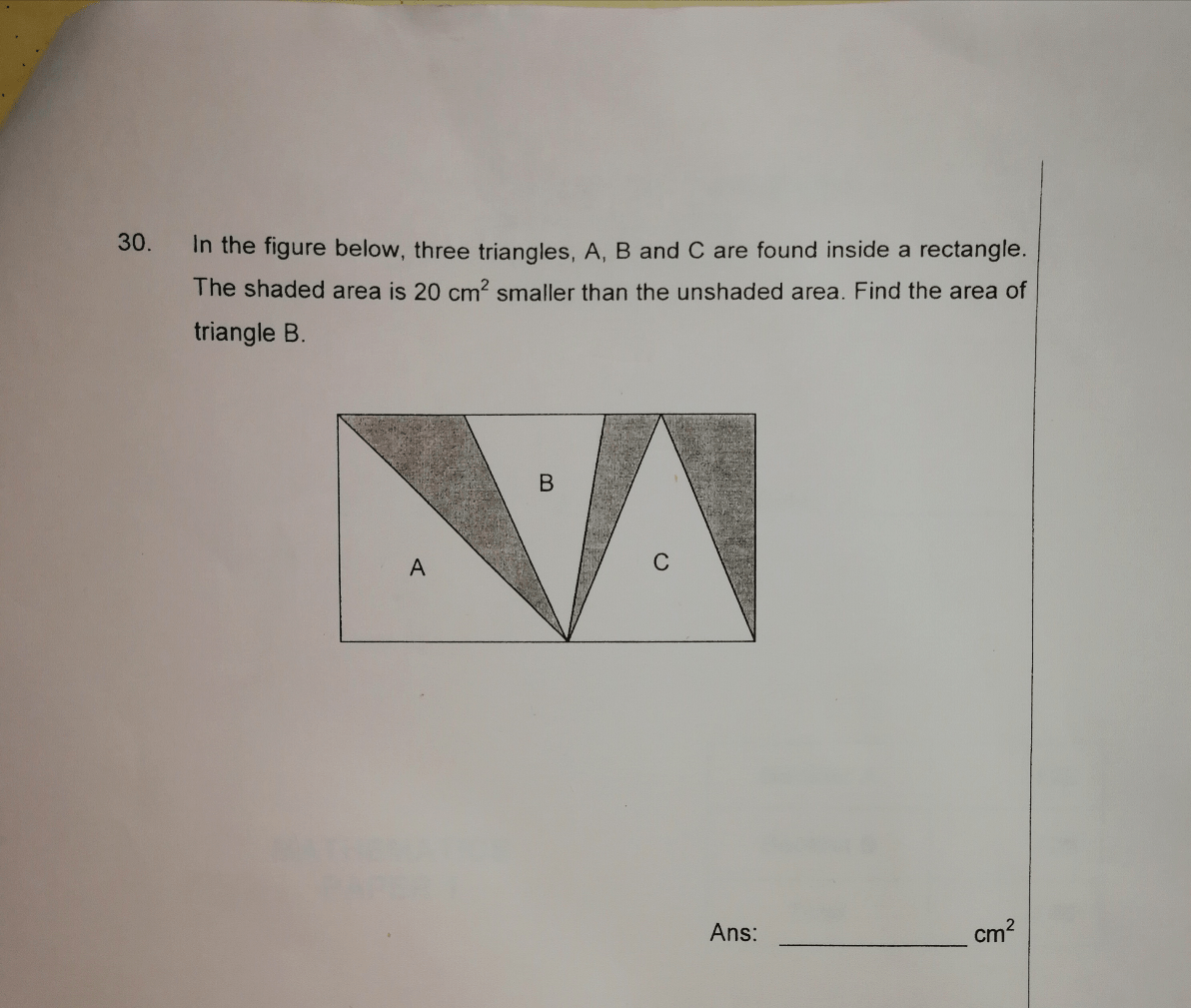# QuestionPlease help with this question.

Source: Fairfield Methodist Primary

1 Answer

# Answer

shaded area + 20 = unshaded area = area of triangle (A + B + C)
shaded area = area of triangle (A + B + C) – 20
shaded area + area of triangle B = area of triangles (A + C) (same bases and same heights)
area of triangle (A + B + C) – 20 + area of triangle B = area of triangles (A + C)
area of triangle B = 20/2 = 10

Ans : 10 sq cm.

0 Replies 1 Like